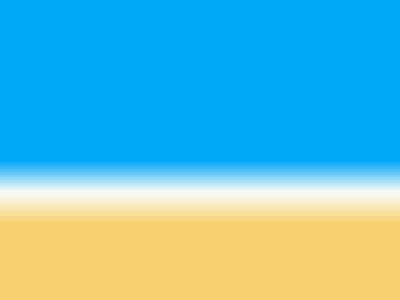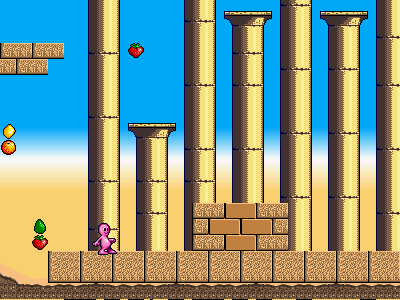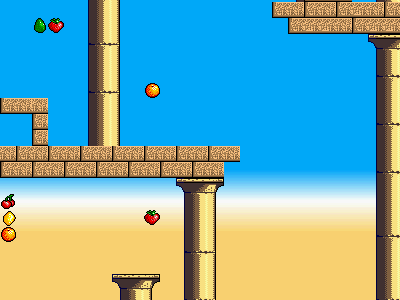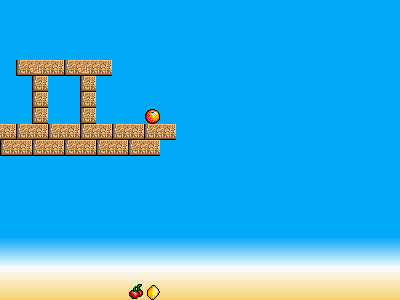# Background color register

Background character colors have a transparency bit. When this bit is set, the hardware takes the color from the BG_COLOR register.

This is useful for situations when a color is shared across the whole background and you want to update it at once - for a flash or fade, for example.

But by using a small coprocessor program (see below) to load the background color register with a new value for each raster line, you can use it like another graphic layer behind the character graphics.

The microprogram reads a palette of 64 colors from memory, and draws them over 64 raster lines. With a suitable desert-themed 64 color gradient (blue to white to sand) loaded, the result is:The video above is this background gradient, plus:

• character graphics for the scrolling background image of blocks and columns
• 16-color sprites for the standing character and fruit

The parallax scroll gives an impression of height: the gradient slowly moves down as the camera climbs up. On the ground the gradient is placed quite low to make a horizon:higherhigher still...```#include <SPI.h>
#include <GD.h>

int atxy(int x, int y)
{
return (y << 6) + x;
}

#include "bg.h"
#include "bgstripes.h"

class GDflashbits {
public:
void begin(prog_uchar *s) {
src = s;
}
byte get1(void) {
src++;
}
return r;
}
unsigned short getn(byte n) {
unsigned short r = 0;
while (n--) {
r <<= 1;
r |= get1();
}
return r;
}
private:
prog_uchar *src;
};

static GDflashbits GDFB;

static void GD_uncompress(unsigned int addr, PROGMEM prog_uchar *src)
{
GDFB.begin(src);
byte b_off = GDFB.getn(4);
byte b_len = GDFB.getn(4);
byte minlen = GDFB.getn(2);
unsigned short items = GDFB.getn(16);
while (items--) {
if (GDFB.get1() == 0) {
} else {
int offset = -GDFB.getn(b_off) - 1;
int l = GDFB.getn(b_len) + minlen;
while (l--) {
}
}
}
}

static void setup_sprites()
{
GD.copy(PALETTE16A, palette16a, sizeof(palette16a));
GD.copy(PALETTE16B, palette16b, sizeof(palette16b));
GD_uncompress(RAM_SPRIMG, sprimg_cc);

GD.wr(JK_MODE, 1);
}

static int shot = 0;

void setup()
{

GD.begin();
setup_sprites();
GD_uncompress(RAM_CHR, dchr);
GD_uncompress(RAM_PAL, dpal);
GD.microcode(bgstripes_code, sizeof(bgstripes_code));
GD.copy(0x3e80, desert, sizeof(desert));

int ypos;
int delta = 1;
int speedup = 0;
for (ypos = 2048 - 299; ypos > 0; ypos -= delta) {
if (++speedup == 90) {
speedup = 0;
delta++;
}
GD.__wstartspr(0);
int jy = (2048-44) - ypos;
if (jy < 400)
draw_walk(100, jy, 0, 0);
GD.__end();

int yd = ypos >> 4;

GD.wr16(SCROLL_Y, ypos & 15);
GD.wr(COMM+0, 236 - yd);
int x, y;
int fruit = 64;
for (y = 0; y < 32; y++) {
for (x = 0; x < 32; x++) {
byte t = pgm_read_byte_near(level + ((y + yd) << 5) + x);
if (t == 4) {
GD.__wstartspr(fruit);
draw_fruit(16 * x + 8, 16 * y - (ypos & 15) + 8, ((x + y + yd) % 6), 0);
GD.__end();
fruit++;
t = 0;
}
PROGMEM prog_uchar *pt = tiles + (t << 2);
int da = (x << 1) + (y << 7);
GD.copy(da, pt, 2);
GD.copy(da + 64, pt + 2, 2);
}
}

// GD.screenshot(shot);
shot++;
}
}

void loop()
{
}
```

# bgstripes.fs

```start-microcode bgstripes

\ renders a 64-line horizontal stripe in the BG_COLOR
\ starting at line COMM+0

\ Interface:
\ COMM+0    stripe start
\ 3E80-3EFF 64 color stripe

: 1+    d# 1 + ;
: -     invert 1+ + ;
: 0=    d# 0 = ;
: @     dup c@ swap 1+ c@ swab or ;
: !     over swab over 1+ c! c! ;
: 2dup  over over ;
: min   2dup < ;fallthru
: ?:    ( xt xf flag -- xt | xf)    \ if flag xt, else xf
if drop else nip then ;
: max   2dup swap < ?: ;

: main
begin
YLINE c@            \ line COMM+0 is line zero
COMM+0 c@ -
d# 0 max d# 63 min  \ clamp to 0-63
d# 2 * h# 3E80 +    \ index into color table
@ BG_COLOR !        \ fetch and write
again
;

end-microcode
```